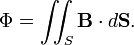# Magnetic flux

In physics, magnetic flux Φ is the total magnetic induction B summed or integrated over a surface S. This explains the alternative name magnetic flux density (magnetic flux per unit of surface) for the magnetic induction B.

If B is constant over S, Φ is simply equal to B times the area of S. If B varies across S, Φ is the integral$\Phi = \iint_S \mathbf{B}\cdot d\mathbf{S}.$

The integrand (expression under the integral) is an inner product of the vector B and the vector dS. The latter vector has the magnitude of the infinitesimal surface element dS and has the direction of the normal n (unit vector perpendicular to the surface element) on dS. The normal n can be pointing from the "top side" or from the "bottom side" of dS. Which of the two directions n takes, is determined by the context. For instance, when S is part of closed surface, enveloping a volume, then n points to the outside of the volume.

Magnetic flux obeys Gauss' law.

The SI unit for magnetic flux is the weber (symbol Wb). The Gaussian unit for magnetic flux is the maxwell (symbol Mx). 1 Mx = 1 gauss⋅cm2 = 10−8 Wb.